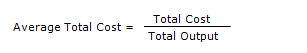Meaning
Cost of production refers to the prices paid by the firm in order to obtain the materials and services required for the production process.

There are two types of costs.

1. Variable Costs
• It refers to the cost of variable factors
• It changes with output
• There is no variable cost at zero output
• It is also known as Direct Costs
• Examples include Electricity bill, cost of raw materials etc.

2. Fixed Costs
• It refers to the cost of fixed factors
• It does not change with output
• It occurs at zero output
• It is also known as Indirect Costs
• Examples include Rent for the factory, Insurance Premium etc.

Total Cost
The sum of variable cost and fixed cost forms the Total Cost.
Total Cost = Variable Cost + Fixed Cost

Marginal Cost
It is the change in the total cost brought about by changing the output by one unit.

Average Total Cost
It is the cost per unit of output.Average Fixed Cost
It refers to the Fixed Cost per unit of output.

Average Variable Cost
It refers to the Variable Cost per unit of output.##### Ahmed Xahir

This is a short biography of the post author. Maecenas nec odio et ante tincidunt tempus donec vitae sapien ut libero venenatis faucibus nullam quis ante maecenas nec odio et ante tincidunt tempus donec.